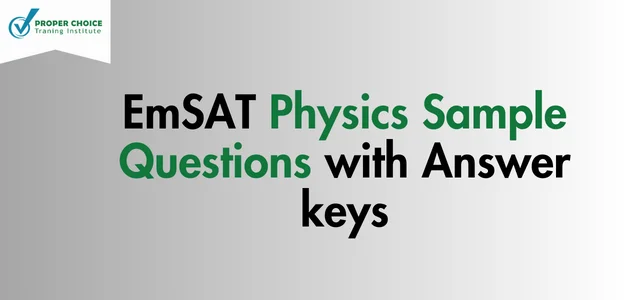# EmSAT Physics Sample Questions with Answer Keys – 2023Prepare for the EmSAT Physics exam in 2023 with our comprehensive collection of EmSAT Physics sample questions and answer keys. Boost your preparation and increase your chances of success. Don’t miss out on this valuable resource. Get started today!

Question 1

A block of mass 5 kg slides down a frictionless inclined plane that makes a 40°angle with the horizontal. Find the acceleration of the block.

• A) 5 mls2
• B) 6 m/s2
• C) 6.3 m/s2
• D) 7. 5 m/s2

Question 2

How much tension a rope must have to lift a 100 N object with an acceleration of 8 m/s2 (g = 9.8 m/s2)

• A) 200 N
• B) 182 N
• C) 150 N
• D) 100 N

Question 3

A crate of mass 150 kg rests on the floor. The coefficient of statics friction is 0.35. If the force of 200 N, parallel to the floor is applied to the crate, what is the magnitude of the force of static friction on the crate?

• A) 514. 5 N
• B) 437.5 N
• C) 380 N
• D) 200 N

Question 4

A woman riding a bicycle collides head – on with a parked school bus. Which object feels the greater force?

• A) Woman
• B) Bus
• C) Both woman and bus feel equal force
• D) Cannot say anything

Question 5

A box slides down an inclined plane (incline angle is 40°). The mass of the box is 50 kg and the length of the ramp is 10 m. How much is the work done by gravity?

• A) 3149.65 J
• B) 3753.61 J
• C) 3945.47 J
• D) 4000 J

Question 6

A skydiver jumps from a hovering helicopter that is 2000 m above the ground. If air resistance can be ignored, how fast will he be falling when his altitude is 750 m?

• A) 140.80 m/s
• B) 156.52 m/s
• C) 179.83 m/s
• D) 183.49 m/s

Question 7

What must be the power output of a rocket engine, which moves a 2000 kg rocket at a constant speed of 6.0 m/s?

• A) 120.54 kW
• B) 190.86 kW
• C) 117.60 kW
• D) 109.43 kW

Question 8

The angular velocity of a rotating disk increases from 3 rad/s to 8 rad/s in 0.7 s. What is the disc’s average angular acceleration?

Question 9

A block of mass m = 0.03 kg oscillates on a spring whose force constant is 800 N/m. The amplitude of the oscillation is 5.0 cm. The maximum speed of the block is?

• A) 8 m/s
• B) 9 m/s
• C) 10 m/s
• D) 11 m/s

Question 10

A simple pendulum has a period of 1 s on the earth. What would be its period on the Moon (where g is one -sixth of its value here)?

• A) 1. 8 s
• B) 2.4 s
• C) 3.0 s
• D) 6.0 s